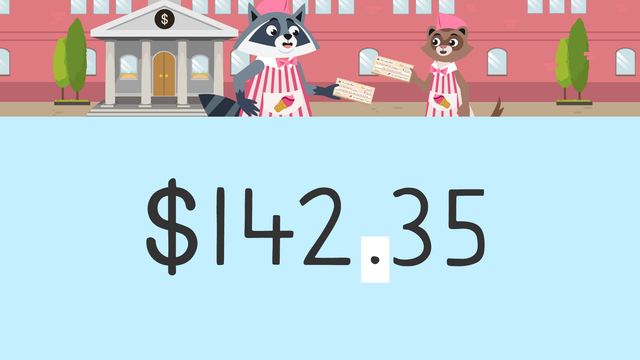# Decimal and MoneyRating

Ø 5.0 / 1 ratings
The authorsTeam Digital

## Decimals and Money – Introduction

Have you ever looked at a grocery bill? When you look at the monetary amounts, you will often see something like \$3.52. This means that the price is between 3 dollars and 4 dollars. With decimal numbers like 3.52 you can indicate amounts between whole numbers. Did you know a decimal number is often represented as money? This is explained in our video lesson about money with decimal points. After the video, you can also find exercises and worksheets about money with decimals for fourth grade.

## Decimals and Money – Place Value Chart

If you are not quite sure about money and decimals yet, have a look at the place value chart below. It can help you understand money in decimal form.In this place value chart you can see an amount of money with decimal places. The hundreds place represents one hundred dollars, the tens place represents ten dollars, and the ones place represents one dollar. The decimal point separates dollars and cents. The tenths place represents ten cents and the hundredths place represents one cent.

## Decimals and Money – Example and PracticeIn this place value chart, showing a decimal number as money, there are no hundreds. Therefore, we start with the tens place value. There are sixty dollars in the tens place and three dollars in the ones place. For the cents, there are forty cents in the tenths place and two cents in the hundredths place. This means there are sixty-three dollars and forty-two cents in total, which is the same as sixty dollars plus three dollars, plus forty cents, plus two cents.

T O . T H
6 3 . 4 2

## Decimals and Money – Summary

If you want to practice money with decimal places on your own, check out our interactive exercises and worksheets on decimals and money.

### TranscriptDecimal and Money

Zuri and Freddie have recieved their first pay check and need to deposit them! Upon closer inspection of their checks, they realize they don't know how much money they made because they do not understand the value of each digit. Let's help Zuri and Freddie learn all about place value, decimals and money. Dollars and cents are separated by a decimal. A place value chart can help to understand the relationship between decimals and money! The hundreds place represents one hundred dollars. The tens place represents ten dollars. The ones place represents one dollar. The decimal point separates the dollars from cents. We say and to represent the decimal point when talking about money. The tenths place can be thought of as one dollar divided into ten parts, and each part is equal to ten cents. The hundredths place can be thought of as one dollar divided into one hundred parts, and each part is equal to one cent. Let's look at an example. Freddy's paycheck has sixty-three dollars and forty-two cents. There are no hundreds, so start with the tens place value. The tens place value has a six, which represents six ten dollar bills or sixty dollars. The ones place value has a three which represents three one dollar bills, or three dollars. Now let's look at the decimal place values where cents represent parts of a whole dollar. The tenths place value has a four, which represents four ten cent coins, or forty cents. The hundredths place value has a two, which represents two one cent coins, or two cents! Sixty-three dollars and forty-two cents is the same as sixty dollars, plus three dollars, plus forty cents, plus two cents! Now that we have looked at place value, decimals, and money, let's help Zuri with her pay check! Her pay check has one hundred forty-two dollars AND thirty-five cents written on it. What is the value of the hundreds place? There is a one, which represents one hundred dollars. What is the value of the tens place? There is a four, which represents forty dollars. What is the value of the ones place? There is a two, which represents two dollars. What is the value of the tenths place? There is a three, which shows thirty cents. What is the value of the hundredths place? There is a five, which represents five cents. Since five pennies is equal to one nickel, we could also use a nickel here instead! One hundred forty-two and thirty-five cents is the same as one hundred dollars, plus forty dollars, plus two dollars, plus thirty cents, plus five cents! While Freddie and Zuri cash in their checks, let's review. Remember, a decimal separate dollars from cents. The hundreds place represents one hundred dollars. The tens place represents ten dollars. The ones place represents one dollar. The tenths place represents ten cents. The hundredths place represents one cent. "What should we do with our money?" "Shall we get ice cream?" "Yes a thousand times!" "Freddie, isn't that the ice cream truck that we work at?"